# LOG#093. Group theory(XIII).

The sixtor or 6D Riemann-Silberstein vector is a complex-valued quantity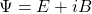up to one multiplicative constant and it can be understood as a bivector field in Clifford algebras/geometric calculus/geometric algebra. But we are not going to go so far in this post. We remark only that a bivector field is something different to a normal vector or even, as we saw in the previous post, a bivector field can not be the same thing that a spinor field with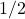spin. Moreover, the electric and magnetic parts of the sixtor transform as vectors under spatial rotations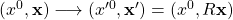where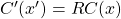and R being an orientation preserving rotation matrix in the spcial orthogonal group. Remember that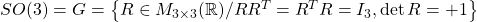The groupis bases on the preservation of the scalar (inner) product defined by certain quadratic form acting on 3D vectors:andsofor proper rotations. This excludes spatial reflections or parity transformations P, that in fact are important too. Parity transformations act differently to electric and magnetic fields and they have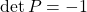. Parity transformations belong to the group of “improper” rotations in 3D space.

However, the electromagnetic field components are NOT related to the spatial components of a 4D vector. That is not true. With respect to the proper Lorentz group: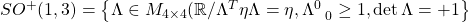and where the metricis the Minkovski metric. In fact, the explicit representation of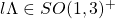by its matrix elements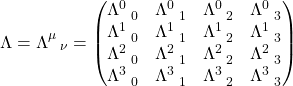Despite this fact, that the electromagnetic field sixtor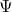transforms as a vector under the COMPLEX special orthogonal groupin 3D space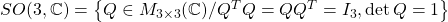This observation is related to the fact that the proper Lorentz group and the complex rotation group are isomorphic to each other as Lie groups, i.e.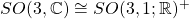. This analogy and mathematical result has some deeper consequences in the theory of the so-called Dirac, Weyl and Majorana spinors (quantum fields describing fermions with differnt number of independent “components”) in the massive case.

The puzzle, now,  is to understand why the mass term is forbidden in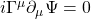as it is the case of the electromagnetic (classical) field. Moreover, in this problem, we will see that there is a relation between symmetries and operators of the Lie groupsand the corresponding generators of their respective Lie algebras. Let’s  begin with pure boosts in some space-time plane: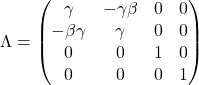and where we defined, as usual,and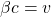with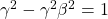.

Then,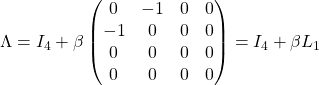and where we have defined the boost generator as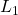in the plane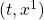, and the boost parameter will be given by the numberRemark: Lorentz transformations/boosts corresponds to rotations with an “imaginary angle”.

Moreover, we also getEquivalently, by “empathic mimicry” we can introduce the boost generators in the remaining 3 planesas follows:In addition to these 3 Lorentz boosts, we can introduce and define another 3 generators related to the classicla rotation in 3D space. Their generators would be given by: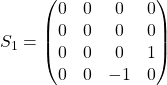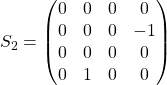Therefore,andspan the proper Lorent Lie algebrawith generators. These generators satisfy the commutators: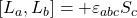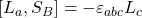withandthe totally antisymmetric tensor. This Levi-Civita symbol is also basic in thestructure constants. Generally speaking, in the Physics realm, the generators are usually chosen to be hermitian and an additional imaginarfactor should be included in the above calculations to get hermitian generators. If we focus on the groupover the real numbers, i.e., the usual rotation group, the Lie algebra basis is given by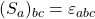, or equivalently by the matrices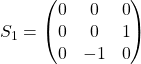and the commutation rules areIf the rotation matrix is approximated to: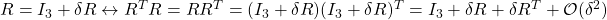then we have that the rotation matrix is antisymmetric, since we should haveorso the matrix generators are antisymmetric matrices in theLie algebra. That is, the generators are real and antisymmetric. The S-matrices span the fullreal Lie algebra. In the same way, we could do the same for the complex groupand we could obtain the Lie algebraover the complex numbers with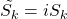added to the realof thereal Lie group. This defines a “complexification” of the Lie group and it implies that: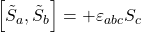Do you remember this algebra but with another different notation? Yes! This is the same Lie algebra we obtained in the case of the Lorentz group. Therefore, the Lie algebras of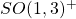andare isomorphic if we make the identifications: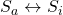The rotation part ofis the rotation part ofThe boost part ofis the complex conjugated (rotation-like) part ofThen for every matrix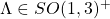we haveand for every matrix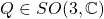we have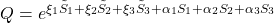For instance, a bivector boost in some axis provides:and where in the first matrix, it acts on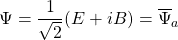, the second matrix (as the first one) acts also on a complex sixtor, and where the rotation around the axis perpendicular to the rotation plane is defined by the matrix operator: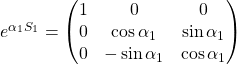and this matrix would belong to the real orthogonal group.

Note: Acting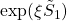onto the sixtoras a bivector field shows that it generates the correct Lorentz transformation of the full electromagnetic field! The check is quite straightforward, since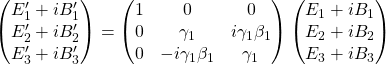From this complex matrix we easily read off the transformation of electric and magnetic fields: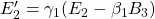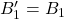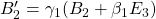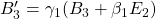Note the symmetry between electric and magnetic fields hidden in the sixtor/bivector approach!

For the general Lorentz transformationwe have the invariantsThis site uses Akismet to reduce spam. Learn how your comment data is processed.